# Measuring the Rheological Behavior of Polymer Solutions

Polymers are versatile materials that play an important role in a plethora of industrial applications. Polymeric solutions or suspensions provide joint lubrication in the human body, make foods appear rich and creamy and impart stability to inks and paints. In most applications, the rheological characteristics of polymers has an impact on both product functionality and performance.

Rheology is defined as the study of material deformation and flow. Based on rheology the characteristics of polymers such as their molecular size, structure and molecular weight (MW) can be directly linked with product performance. Defining rheological targets in terms of viscosity or viscoelasticity in order to attain performance goals is an efficient formulation strategy. However, the success of this strategy depends on the measurement of relevant rheological data.

This article evaluates the main rheological characteristics of polymer solutions and various measurement techniques for these characteristics. Microrheology and rotational and microfluidic rheometry are some of the techniques discussed here. The case studies highlight the data generated and potential applications.

## Role of Rheology in Formulation

Formulators generally apply two strategies that are complementary while defining product performance targets. The first strategy works towards altering the composition of the formulation whilst the second changes the physical characteristics of the constituents.

In formulations containing polymers the polymer concentration and molecular weight are the two variables that are altered most. However, there are numerous factors other than those that affect the polymer behavior. Some of these characteristics are temperature, polymer charge and solvent quality as well as the type and structure of the polymer.

Typically, formulation is an empirical process that is performed by altering the formulation and examining the impact of the changes through the measurement of product performance. This method is time consuming and does not provide an understanding of the correlation between the change and the observed effect. Also, correlating the test results with a measurable difference in the various grades available may be difficult. Formulations done this way do not provide substantial information for refining the choice of ingredients.

Rheology can help correlate the formulation changes with the product performance. Knowledge of the yield stress and viscoelastic profile of a product that has a good demand on the market will help in setting up rheological targets for the formulation of a new product to be launched in the same market. Product performance metrics that are difficult to measure can be substituted by these rheological targets. Though the rheology-based approach is faster and fact-based there is a dependency on the measurement of rheological properties.

Apart from being used as thickeners, polymers solutions can also be used to induce viscoelasticity. A system that behaves as a liquid (viscous) and a solid (elastic) is deemed viscoelastic. Viscoelastic behavior supports the performance of numerous consumer products.

## Understanding the Intrinsic Behavior of Polymers in Solution

The volume of the dispersed phase within a solution or a suspension controls its rheology. The viscosity is increased by dispersed particles and the friction present at the surface of the particle disrupting the flow lines.

The relation between the loading of solids and viscosity for dilute systems is given by Einstein’s equation for hard spheres. In the equation, η represents the viscosity of the suspension, ηmedium represents the viscosity of the medium and Φ is the volume fraction of solids present in the suspension.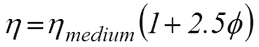The linear relationship suggests that an increase in the number of particles present in the solution causes a proportional increase in the viscosity. This equation is also applicable for polymer solutions in which the polymer is an impenetrable sphere and restricts the flow of solvent. The below equation is a rearranged form of Einstein's equation in which the volume fraction is converted to concentration using the molecular density and intrinsic viscosity (IV). In the equation η stands for the viscosity of the dispersion, ηmedium for medium’s viscosity, c for the concentration of polymer and [η] for the intrinsic viscosity of the polymer in the specific solvent.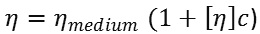Intrinsic viscosity is measured in terms of volume per unit mass and is inversely proportional to the molecular density. Therefore, a polymer molecule with lesser density will exhibit higher value of IV. The volume occupied by the polymer determines its effect on the viscosity of the solution. Therefore, a polymer with a higher IV, higher volume per unit mass, will affect the viscosity of the solution more than a polymer with low IV, provided all other conditions remain the same. Using equation 2 and the IV value, the viscosity of a polymer solution can be measured for dilute solutions, i.e. concentrations below 10%.

## Determining the Value of Intrinsic Viscosity (IV)

The intrinsic viscosity and the molecular weight of the polymer are related, but other structural parameters like the degree of branching of the polymer chain and the type of solvent in which the polymer is dissolved also impact the intrinsic viscosity.

The relationship of IV with these parameters is given by the Kuhn-Mark-Houwink Sakurada equation or simply the Mark-Houwink (M-H) equation. This equation can be used to calculate the IV value for a specific polymer in any solution, if the M-H parameters of K and α are known. These parameters are available in most literature regarding polymer/solvent systems.

The IV value can also be measured by combining the Huggins or Kraemer equations with values from rotational or microfluidic rheometric methods that measure the viscosity over a wide range of concentrations. In the Huggins or Kraemer equations given below, [η] is the intrinsic viscosity; Mw is the molecular weight; and K and α are the M-H parameters.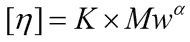The volume occupied by the polymer can also be defined in terms of the radius of gyration (Rg); the value of Rg is given by the average squared distance between any point in the polymer coil and its center of mass.

Figure 1 shows the determination of Rg through light scattering. The value of Rg can be determined directly by light scattering and bears a direct relation with IV as a function of polymer MW. The IV value of different polymer solutions can be calculated and compared based on the measured value of Rg.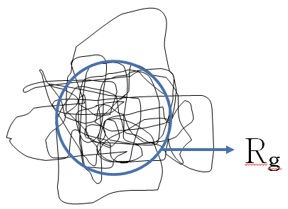Figure 1. Radius of gyration (Rg), which can be determined from light scattering data, is a useful measure of the size of a polymer coil.

Particularly in dilute solutions the influence on the viscosity of a polymer coil is controlled by the volume occupied by it, which is similar to its effect in non-polymeric solutes. Since the volume occupied by a polymer depends on the IV or molecular density the measurement of the Rg value provides the value of IV.

In the case of highly concentrated polymer solutions the polymer coils start interacting with one-another. At this juncture, the Einstein model starts breaking down and the solution’s behavior becomes considerably more complex.

## Onset of Viscoelasticity for Polymers in Solution

The presence of adequate space in a dilute solution eliminates any interaction between individual polymer coils. The viscoelasticity of dilute solutions is solely due to the properties of the individual polymer coils. The overall viscoelasticity of a dilute solution is the product of the viscoelasticity of each coil and the total number of coils. The Rouse-Zimm model illustrates this behavior of polymer coils.

The consequence of increasing the concentration of the polymer coils above the upper limit that defines a dilute solution (~10%) and when the total volume occupied by the coils exceeds the available space is shown in Figure 2.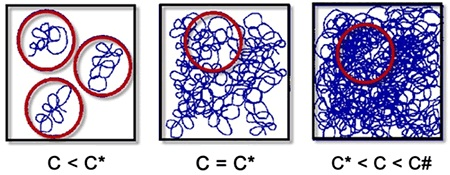Figure 2. As the concentration of polymer coils within a solution increases, coils begin to interact and entangle.

At this point the polymer coils cease to remain separated and tend to entangle. The concentration at this point is called the critical concentration (c*) and its value can be evaluated from Rg or IV measurements due to the relationship between these properties and the volume occupied by the polymer.

The different regimes that occur in polymeric solutions can be described as dilute (c<c#) semi-dilute (c*<c<c#) and concentrated (c>c#). The overlap concentration can be estimated by determining the point at which the product of the number of coils per volume (v) and the volume occupied by a single coil (Rg3) is equal to one using the following equation. Rg is the radius of gyration; Mw is the molecular weight and NA is Avogadro’s constant.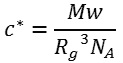An alternate approach is to use the inverse value of IV as an approximate value of c*. However, this approach provides lower values. Beyond c* the viscosity of a polymer solution depends more on the concentration, as illustrated by Figure 3.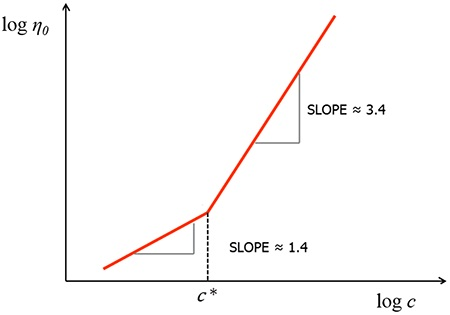Figure 3. Expected zero shear viscosity profiles for polymer solutions above and below c*.

The logarithmic plot is a gradient of 3.4 above c* compared to a gradient of 1.4 below. This is because beyond c* the rheological behavior of the system is controlled by interactions between multiple coils instead of the properties of individual polymer molecules.

A viscous drag occurs due to the increase in polymer interaction. Similar plots can help in estimating c* and also provide values that are in line with values calculated using Rg. The transition between the three regimes is governed by the viscoelastic properties of the polymer solution. In a viscoelastic system the frequency or the timescale for which the shear force is applied is an important factor that determines the rheology and it defines the dominance of elastic or viscous behavior (Figure 4).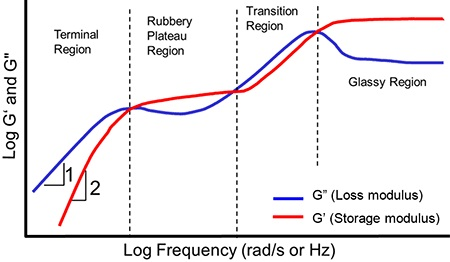Figure 4. The viscoelastic behavior of polymer solutions is a function of the timescale (inverse frequency) over which shear is applied.

The Rouse-Zimm behavior is exhibited by comparatively concentrated polymer solutions at longer timescales (or lower frequencies) and is observed in dilute solutions where the value of the loss modulus (G") is more than the elastic modulus (G'). In this scenario, the polymer chains have adequate time to disentangle and slip by each other by the process of ‘reptation’. A ‘rubbery plateau’ region occurs at higher frequencies where G' is more dominant than the G".

These timescales allow polymer chains to come out of the entanglements that cause tension in the chain segments between the coupling points, and hence, the elastic behavior dominates. The length of the rubbery plateau region relates to the MW of the polymer and its concentration. The value of the angular frequency at which G' and G" intersect at low frequency values is equal to 1/ tmax, where tmax is the longest relaxation time or the time needed for around 63% of the stored elastic energy to be converted into viscous energy and dissipation.

For shorter timescales (or faster frequencies) a phase transition of the polymers in solution occurs and they tend to exhibit glassy behavior. At this point the polymer becomes predominantly immobile, except for the localized rotational and vibrational deformation modes.

Microrheology and rotational and microfluidic rheometry are essential techniques as there is a need to measure rheology over varied conditions in order to acquire data that is relevant to a particular application of an individual polymer solution. In the case of polymeric solutions with low molecular weight and low concentration critical dynamics can occur on shorter timescales so measurements must be taken at higher frequencies.

## Evaluating the Rheological Toolkit for Polymer Solutions

The working principle of all rheological methods is measurement of the correlation between the applied stress or force and the resulting movement or deformation of the material. The way in which stress is applied varies with each method hence each technique provides different ways of evaluating rheological behavior such as the viscoelasticity under different conditions.

## Rotational Rheometry

The rotational rheometer is a versatile rheological tool that can be configured for several test methods. The most useful tests for polymer solutions are tests that generate simple viscosity flow curves and oscillatory tests that quantify viscoelasticity.

In the rotational rheometer, loading of the sample is done between two plates, or a cone and a plate, or a cup and a bob. Application of a torque on the top plate causes a rotational shear stress on the material and the value of the resulting strain rate (shear rate) or the strain is measured. The testing depends on applied uni-directional rotational shear, or a sinusoidal applied stress pattern as in oscillatory testing.

Rotational rheometers that are currently used have been optimized to provide precisely controlled testing over many decades of torque, enabling measurements over various sample types such as weak structured fluids, pastes and gels.

Measurement capabilities of rotational rheometers are curbed at high shear regions with shear rates ranging from 1000 to 10,000s-1  on the type of sample. These limitations may be caused by sample fracture and subsequent generation of elasticity under turbulence, rotational shear or shear heating.

Such limitations may cause errors in the assessment of the performance of polymer solutions under relevant conditions like inkjet printing or spraying applications. Additionally, oscillatory measurements at very high frequencies may be inhibited by mechanical stabilities.

## Microfluidic Rheometry

Microfluidic rheometry is a relatively new concept in rheological measurements. It is similar to capillary rheometry, and is particularly useful in characterizing polymeric solutions with low viscosity and subjected to very high shear stress rates that exceed 1,000,000s-1. This technique can be used for the analysis of samples where the rotational rheometry technique may not be suitable e.g. drinks, personal care products and inkjet inks.

Using the microfluidic rheometry technique, sharper differentiation of low viscosity samples is possible when compared to rotational rheometry techniques. Microfluidic rheometry also works under high shear rate conditions.

In this technique, the sample is sent through the microfluidic channel and the consequent drop in pressure is measured with the help Micro Electro Mechanical Systems (MEMS) pressure sensors that are placed inside the channel.

MEMS pressure sensors are capable of precise measurement of pressure and require only minute amounts of sample for measurement. Microfluidic rheometry is useful in the study of extensional rheology – the behavior of a fluid when it is stretched through a rectangular microfluidic channel with a parabolic contraction that speeds up and extends the fluid through the contraction.

## Microrheology

Microrheology is an upcoming technique that is being explored by researchers for rheological characterization. Unlike mechanical rheological measurement methods, microrheology determines the rheological properties of a fluid based on the motion of the probe particles within it.

Brownian motion causes the movement of small particles in the fluid, and this motion can be tracked by light scattering techniques. The data from light scattering methods can be used to evaluate the viscoelastic properties. This is the working principle of the dynamic light scattering (DLS) microrheology technique. Since the DLS technique does not depend on the application of an external mechanical stress, it can be used in evaluating polymer solution samples with low viscosity and those that are weakly structured and strain sensitive.

Microrheology is extremely effective when used at the ultra-high frequencies that are required for measuring critical short timescale dynamics of polymer solutions. Viscoelastic profiles of smaller sample volumes can be measured over a broad frequency range and for much shorter timescales using a microrheology technique compared to rotational rheometry.

All these techniques can be combined and used in a systematic way to evaluate the rheological performance of polymer solutions, based on the specific conditions required for various industrial applications. The following case studies illustrate this approach.

## Case Study 1: Measuring the Concentration Dependence of Polymer Solution Viscosity

The viscosity profiles for a group of polymer solutions with increasing concentrations are shown in Figure 5. The concentration of these solutions was more than the critical overlap concentration. The value of shear viscosity was determined as a function of the shear rate for each of the solutions, over a broad range of shear rates using Malvern Panalytical’ Kinexus rotational rheometer.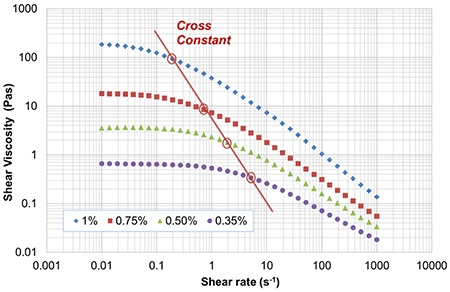Figure 5. Viscosity profiles for polymer solutions of increasing concentration measured using a rotational rheometer.

A constant shear viscosity plateau was observed at low shear rates, which was termed as the zero shear viscosity (η0). Disruption of the entangled polymer coils happens very slowly in this region, causing simultaneous re-entanglement. The re-entanglement results in zero net change in the extent of entanglement and as a result the viscosity remains the same.

Conversely, at higher shear rates the rate of disruption is more than the rate of re-entanglement making the solution less viscous and shear thinning can be observed. The transition to shear thinning takes place at lower shear rates in the case of more concentrated solutions due to a higher entanglement tendency.

## Case Study 2: Investigating Viscoelasticity using Rotational Rheology and Microrheology

Oscillatory testing was performed on a series of hyaluronic acid (HA) solutions all of which had concentrations greater than the critical overlap concentration, as shown in Figure 6. The measurements were made with the Kinexus rotational rheometer.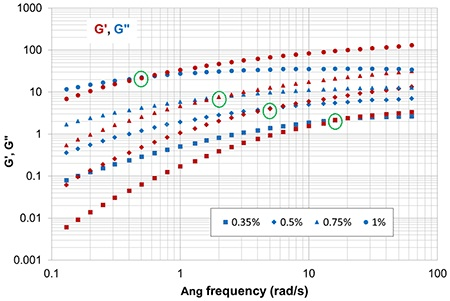Figure 6. Measuring the viscoelasticity of Hyaluronic Acid (HA) using rotational rheometry.

For each of the solutions a well-defined crossover point was observed when G (elastic modulus) was more than the G" (viscous modulus). The generic rheological behavior observed in Figure 4 was reflected in these results where the crossover point signaled the transition to a rubbery state in which the solution exhibited solid-like (elastic) behavior. For lower concentrations the crossover point occurred at higher frequencies and the rubbery state was smaller because of reduced entanglements.

The crossover frequency is a critical value, since it can be used to calculate the longest relaxation time (tmax) of the polymer. The value of tmax is obtained as the inverse value of the angular frequency at the crossover point (Figure 6). The value of tmax tends to increase when molecular weight and concentration is more and is equal to the time taken by the polymer molecules in the solution to disentangle.

Practically, the main factor in defining the behavior of the material is the time the stress is applied relative to the relaxation time of the material. This is defined by the Deborah number (De), where De = tmax /t or ωtmax. Solid-like behavior is exhibited when De>1, and liquid-like behavior when De<1. To extend this study to lower concentrations in the order of 0.1% by weight of the MW HA being measured, the rheological measuring technique needed to be changed in order to overcome the mechanical shortcomings of the rotational rheometer at low concentration/high frequency conditions. Hence, DLS microrheology was used (Figure 7).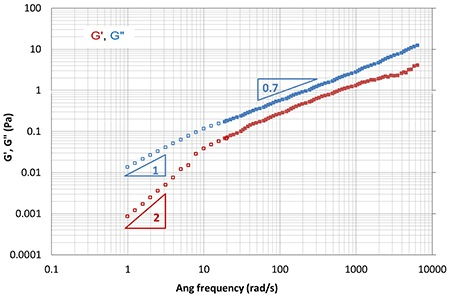Figure 7. Using microrheology it becomes possible to study the viscoelastic behavior of dilute polymer solutions at high frequency.

Measurements were made using Malvern Panalytical’ Zetasizer. Without taking the DLS data into consideration, it may be concluded that G' and G" intersect at increasing frequencies, as depicted in Figure 6. The data obtained from microrheology technique is in contrast to the above conclusion, and that the Rouse- Zimm behavior that describes the behavior of dilute polymeric solutions prevails.

## Case Study 3: Combining Rheological Techniques to Fully Scope the Behavior of Polymer Solutions

Through this study, the behavior of polyacrylamide (HPAM) solution was examined over a broad range of conditions for a comprehensive assessment of rheological performance. The molecular weight of polyacrylamide is relatively high, in the order of 4,000,000, and it formed a relatively high concentrated solution (0.1%w/v), which was above c*. The oscillatory testing method was applied first as shown in Figure 8.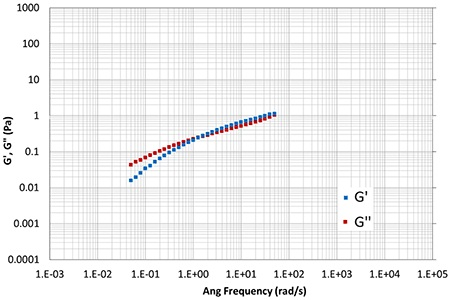Figure 8. For a moderately concentrated HPAM solution rotational rheometry enables measurement in the terminal region and detection of the start of the rubbery plateau.

This testing method is successful in measuring the performance in the terminal regimes and in detecting the beginning of rubbery behavior. Nevertheless, oscillatory testing using the rotational rheometer does not work in certain areas. Although testing at lower frequencies is possible with oscillation testing, there are limitations to the time of the test because a complete oscillation or at least a major portion of the cycle needs to be completed at each frequency which can take a long time. Figure 9 shows the results of creep testing, which is an alternative rotational rheometry method.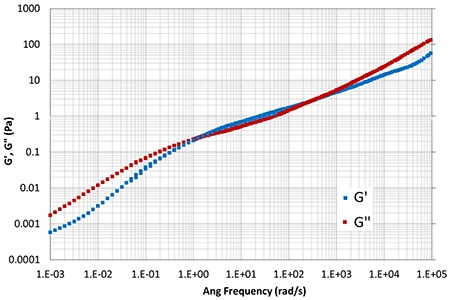Figure 9. Creep testing and microrheology provide complementary data to oscillatory testing to fully scope polymer solution behavior across a very wide range of frequencies.

A creep testing technique is used to evaluate behavior over long timescales/low frequencies. This technique applies a stress on the material for a given period of time and the angular frequency is equal to the inverse of each discrete time point, similar to the DLS microrheology method. Using Kinexus, an algorithm is applied to the creep data using the rSpace software, which converts creep data into G" and G' values.

Microrheology enables access to ultra-high frequency data so it can be used to examine the transition phase beyond the rubbery plateau, which happens before glassy behavior is developed.

Moving beyond viscoelasticity into the simpler concept of viscosity, clarifies the role of microfluidic rheology in evaluating rheological behavior. The viscosity profile of HPAM solution is shown in Figure 10. Rotational rheometry is not capable of providing access to the very high shear rates that are common in industrial processes. For such applications, microfluidic rheometry is a suitable alternative.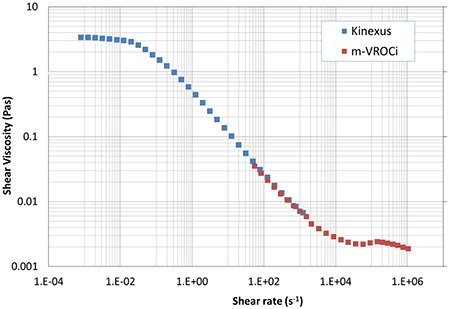Figure 10. Rotational rheometry and microfluidic rheometry provide complimentary viscosity data at low and high shear rates.

## Conclusion

Though rheological behavior of polymeric solutions is complex it is useful in a number of industrial applications. For a complete understanding of their behavior to use polymer solutions formulations most effectively measurements need to be made under appropriate conditions.This information has been sourced, reviewed and adapted from materials provided by Malvern Panalytical.

## Citations

• APA

Malvern Panalytical. (2019, September 03). Measuring the Rheological Behavior of Polymer Solutions. AZoM. Retrieved on January 17, 2020 from https://www.azom.com/article.aspx?ArticleID=12366.

• MLA

Malvern Panalytical. "Measuring the Rheological Behavior of Polymer Solutions". AZoM. 17 January 2020. <https://www.azom.com/article.aspx?ArticleID=12366>.

• Chicago

Malvern Panalytical. "Measuring the Rheological Behavior of Polymer Solutions". AZoM. https://www.azom.com/article.aspx?ArticleID=12366. (accessed January 17, 2020).

• Harvard

Malvern Panalytical. 2019. Measuring the Rheological Behavior of Polymer Solutions. AZoM, viewed 17 January 2020, https://www.azom.com/article.aspx?ArticleID=12366.